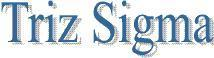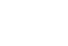Accouting Ratios
Introduction
Accounting Ratio may be defined as the arithmetical expression of the relationship between two accounting figures. For example, gross profit and sales, net profit and sales, sales and total assets, etc.

According to J Betty,  the term accounting ratio is used to describe significant relationships which exist between figures shown in a balance sheet, in a profit & loss account or in a budgetary control system, or in any part of the accounting organisation.

 Does the company have enough cash and current assets to pay obligations as they come due? How efficient are the operations of the company? What is the mix of equity to debt? How profitable is the company? Is it right company to Invest?

Main accounting ratios
 Liquidity Ratios a)      Current Ratio b)      Quick (Liquid/Acid-test) Ratio Profitability Ratios a)      In relation to sales: (i)                  Gross profit Ratio (Gross profit margin) (ii)                Net profit Ratio (Net profit margin) (iii)               Operating profit Ratio (iv)              Operating (Operating cost) Ratio b)      In relation to investment: (i)                  Return on Total Assets (ii)                Return on Capital Employed. (ROCE) Or    Return on Investment (ROI) (iii)               Return on Equity (ROE) Expenses Ratios a)      Administrative Expenses Ratio b)      Selling & Distribution Expenses Ratio Activity Ratios a)      Stock (Inventory) turnover Ratio b)      Stockholding period (Stock velocity) c)      Debtors Turnover Ratio d)      Debt Collection period (Debtors Velocity) e)       Creditors turnover Ratio f)        Debt payment (Creditors Velocity) g)      Working capital turnover Ratio h)      Fixed Assets turnover Ratio i)        Current assets turnover Ratio Solvency Ratios a)      Debt- Equity Ratio b)      Proprietary Ratio c)       Gearing Ratio d)      Interest coverage Ratio Investors Ratios a)      Earning per Share (EPS) b)      Dividend per Share (DPS) c)      Price Earning Ratio (PE Ratio)
a) Simplification of accounting data --- Ratios analysis simplifies and summarizes complex accounting data presented in the trading account, profit & loss account, and the balance sheet. For example gross profit ratio, net profit ratio figures give a better understandable picture of the profitability of a business than the absolute profit figures.

b)Helpful to business managers ---- Ratios can assist managers in performing basic management functions like planning, organising, directing, co-coordinating, budgeting, forecasting, etc.

c)Useful in judging the financial position of a business --- Accounting ratios help in judging liquidity, solvency, profitability, efficiency, etc. of a business. Such an evaluation enables the management to judge the operating efficiency of the various aspects of business.

d) Facilitates inter firm comparison. --- Comparison of the performance of the one firm with another can be made only when the absolute financial figures are converted into the comparable ratios.

e) Makes intra firm comparison possible. --- Ratio analysis makes possible comparison of the performance of the different divisions of the firm.

Limitation of accounting ratios:
a) No suitable standard --- Generally there is no standard ratio for the purpose of the comparison, because conditions of one concern differ significantly from another concern. For example, an ideal current ratio is 2: 1. But if a firm is able to procure additional funds easily and immediately, in that case current ratio of less than 2 may be considered good.

b) No precise terminology --- Terms used to calculate accounting ratios have no standard and precise definition. For example, net profit ratio is calculated by different ways like net profit before tax or net profit after tax.

c) Different accounting policies --- Inter firm comparison is possible provided these firms follow the same accounting policies regarding the method of depreciation, valuation of stock, etc. But this is very rare to see.

d) Window dressing --- To give a better picture of the company, accounting figures may be manipulated.

e) Ratios indicate past performance only --- Ratios are calculated on the basis of past figures. Thus, ratios indicate past performance and not expected future performance.